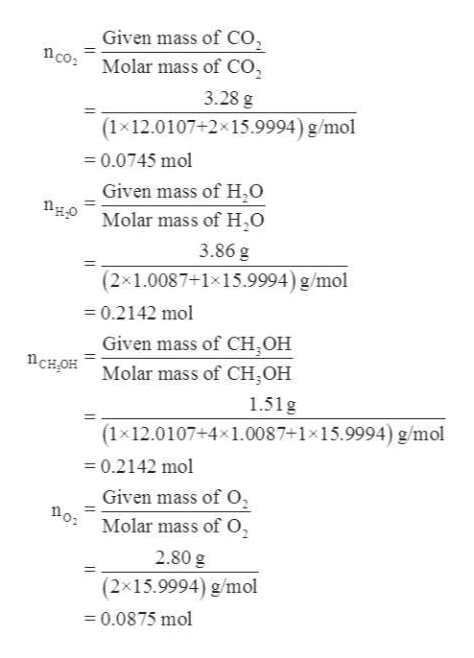# Carbon dioxide and water react to form methanol and oxygen, like this:2CO2(g) + 4H2O(g) → 2CH3OH(l) + 3O2 (g)At a certain temperature, a chemist finds that a 7.5L reaction vessel containing a mixture of carbon dioxide, water, methanol, and oxygen at equilibrium has the following composition:compoundamountCO23.28gH2O3.86gCH3OH1.51gO22.80gCalculate the value of the equilibrium constant Kc for this reaction. Round your answer to 2 significant digits.

Question

Carbon dioxide and water react to form methanol and oxygen, like this:

2CO2(g) + 4H2O(g) → 2CH3OH(l) + 3O2 (g)At a certain temperature, a chemist finds that a 7.5L reaction vessel containing a mixture of carbon dioxide, water, methanol, and oxygen at equilibrium has the following composition:
compound amount
CO2
3.28g
H2O
3.86g
CH3OH
1.51g
O2
2.80g

Calculate the value of the equilibrium constant Kc for this reaction. Round your answer to 2 significant digits.

check_circleExpert Solution
Step 1

It is given that:

Mass of carbon dioxide (CO2) = 3.28 g

Mass of water (H2O) = 3.86 g

Mass of methanol (CH3OH)= 1.51 g

Mass of oxygen (O2) = 2.80 g

Volume of vessel = 7.5 L

Step 2

Equilibrium constant for a reaction can be expressed in terms of concentration of products to the concentration of reactants raised to some power. It is denoted by Kc. The reaction and equilibrium constant for reaction of 2 moles of carbon dioxide with 4 moles of water to give 2 moles of methanol and 3 moles of oxygen is represented as follows:

Step 3

To calculate concentration of a substance number ...help_outlineImage TranscriptioncloseGiven mass of Co Molar mass of CO co 3.28 g (1x12.0107+2 15.9994) g/mol =0.0745 mol Given mass of H,0 HOMolar mass of H,O 3.86 g (2x1.0087+1x15.9994) g/mol 0.2142 mol Given mass of CH OH Molar mass of CH2OH CHOH 1.51g (1x12.0107+4x1.0087+1x15.9994) g/mol -0.2142 mol Given mass of 0 o Molar mass of O 2.80 g (2x15.9994) g/mol 0.0875 mol fullscreen

### Want to see the full answer?

See Solution

#### Want to see this answer and more?

Solutions are written by subject experts who are available 24/7. Questions are typically answered within 1 hour*

See Solution
*Response times may vary by subject and question
Tagged in

### Chemistry